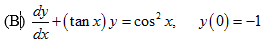# (B) +(tan x) y = cos x, y(0) =-1 dx

Question

Solve the given linear differential equationshelp_outlineImage Transcriptionclose(B) +(tan x) y = cos x, y(0) =-1 dx fullscreen

### Want to see this answer and more?

Experts are waiting 24/7 to provide step-by-step solutions in as fast as 30 minutes!*

*Response times vary by subject and question complexity. Median response time is 34 minutes and may be longer for new subjects.
Tagged in
MathCalculus

### Differential Equations